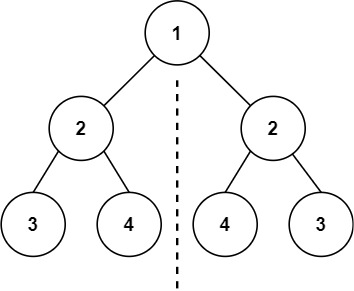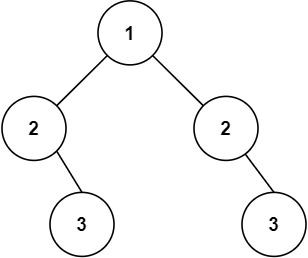# Solved! Leetcode 101. Symmetric Tree

### Symmetric Tree

Given the root of a binary tree, check whether it is a mirror of itself (i.e., symmetric around its center).

Example 1:Input: root = [1,2,2,3,4,4,3]
Output: true

Example 2:Input: root = [1,2,2,null,3,null,3]
Output: false

Constraints:

• The number of nodes in the tree is in the range [1, 1000].
• -100 <= Node.val <= 100

Time complexity:

• O(n), where n is the number of nodes in a tree, as we need to visit every node in the tree

Space Complexity:

• O(1)
Rate this post